Courses

# Previous year questions (2016-20) - Moving Charges and Magnetism (Part - 2) Notes | EduRev

## JEE : Previous year questions (2016-20) - Moving Charges and Magnetism (Part - 2) Notes | EduRev

The document Previous year questions (2016-20) - Moving Charges and Magnetism (Part - 2) Notes | EduRev is a part of the JEE Course Physics Class 12.
All you need of JEE at this link: JEE

Q 26. A paramagnetic substance in the form of a cube with sides 1 cm has a magnetic dipole moment of 20 × 10−6 J/T when a magnetic intensity of 60 × 103 A/m is applied. Its magnetic susceptibility is    
(1) 3.3 × 10−2
(2) 4.3 × 10−2
(3) 2.3 × 10−2
(4) 3.3 × 10−4
Ans:
(4)
Solution: Magnetic susceptibility is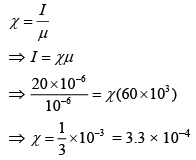Q 27. As shown in the figure, two infinitely long, identical wires are bent by 90° and placed in such a way that the segments LP and QM are along the x-axis, while segments PS and QN are parallel to the y-axis. If OP = OQ = 4 cm, and the magnitude of the magnetic field at O is 10−4 T, and the two wires carry equal currents (see figure), the magnitude of the current in each wire and the direction of the magnetic field at O will be ( μ0 = 4π × 10−7N / A2 )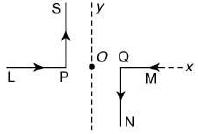(1) 20 A, perpendicular out of the page
(2) 40 A, perpendicular out of the page
(3) 20 A, perpendicular into the page
(4) 40 A, perpendicular into the page
Ans:
(3)
Solution:
Magnetic field at O will be done by PS and QN
Given OP = OQ = 4 × 10−2
Magnetic field at point O is Bnet = 10−4 T
Magnetic field at Point O due to wire LP and MQ is zero.
Let current in each wire = i
Thus, Bnet = BPS + BQN     (both inward)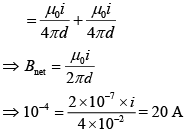Therefore, current is 20 A and it is perpendicular into the page.

Q 28. A proton and an α-particle (with their masses in the ratio of 1 : 4 and charges in the ratio of 1 : 2) are accelerated from rest through a potential difference V. If a uniform magnetic field (B) is set up perpendicular to their velocities, the ratio of the radii rp : rα of the circular paths described by them will be    
(1) 1 : √2
(2)1 : 2
(3) 1 : 3
(4) 1 : √3
Ans:
(1)
Solution:
Kinetic energy = qΔV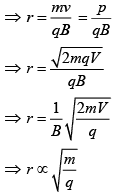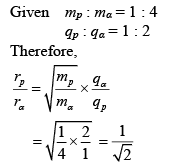Q 29. A paramagnetic material has 1028 atoms/m3. Its magnetic susceptibility at temperature 350 K is 2.8 × 10−4. Its susceptibility at 300 K is    
(1) 3.267 × 10−4
(2) 3.672 × 10−4
(3) 3.726 × 10−4
(4) 2.672 × 10−4
Ans:
(1)
Solution:
where, TC = Curie’s Temperature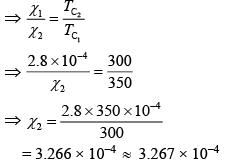Q 30. The mean intensity of radiation on the surface of the Sun is about 108 W/m2. The rms value of the corresponding magnetic field is closest to     
(1) 1 T
(2) 102 T
(3) 10−2 T
(4) 10−4 T
Ans:
(4)
Solution:
Intensity of EM wave is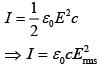As we know that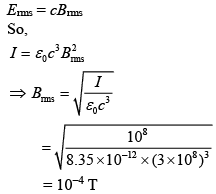Q 31. An electron, a proton and an alpha particle having the same kinetic energy are moving in circular orbits of radii re, rp, rα respectively in a uniform magnetic field B. The relation between re, rp, rα is:    
(1) re > rp = rα
(2) re < rp = rα
(3) re < rp < rα
(4) re < rα < rp
Ans:
(2)
Solution: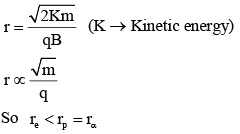Q 32. Suppose that the angular velocity of rotation of earth is increased. Then as a consequence:    
(1) Weight of the object every where on the earth will decrease
(2) Weight of the object every where on the earth will increase
(3) Except at poles weight of the object on the earth will decrease
(4) There will be no change in weight anywhere on the earth
Ans:
(3)
Solution:
g' = g – ω2Rcos2φ
Where φ is latitude there will be no change in gravity at poles as φ = 90°
At all other points as ω increases g' will decrease

Q 33. In a certain region static electric and magentic fields exist. The magnetic field is given by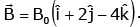If a test charge moving with a velocity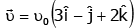experiences no force in that region, then the electric field in the region, in SI units, is -    
(1)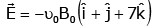(2)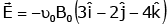(3)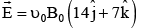(4)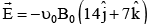Ans:
4
Solution:
Fe = Fn = 0
Fe = -Fm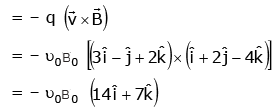Q 34. A uniform magnetic field B of 0.3 T is along the positive Z-direction. A rectangular loop (abcd) of sides 10 cm × 5 cm carries a current I of 12 A. Out of the following different orientations, which one corresponds to stable equilibrium ?    
(1)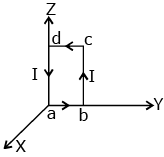(2)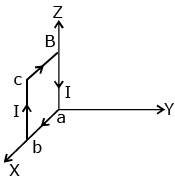(3)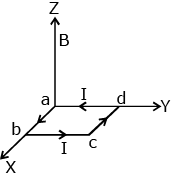(4)Ans: 3
Solution:
For the 3rd option figure,

M = NIA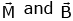are on same direction

Q 35. Two identical wires A and B, each of length  ‘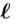’, carry the same current I. Wire A is bent into a circle of radius R and wire B is bent to form a square of side ‘a’. If BA and BB are the values of magnetic field at the centres of the circle and square respectively, then the ratio BA / BB is:    
(1)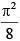(2)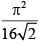(3)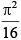(4)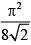Ans:
(4)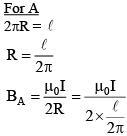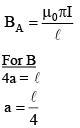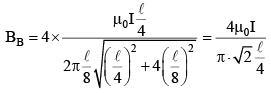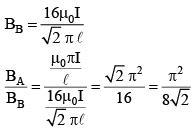Q 36. A conducting metal circular - wire loop of radius r is placed perpendicular to a magnetic field which varies with time as B =B0e–t/τ, where B0 and τ are constants, at t = 0 . If the resistance of the loop is R then the heat generated in the loop after a long time (t →∞) is :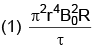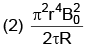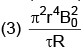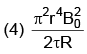Ans: (4)
Solution: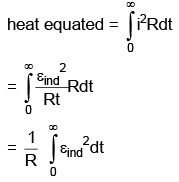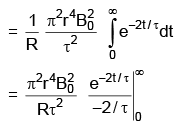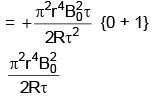Q 37. A fighter plane of length 20 m, wing span (distance from tip of one wing to the tip of the other wing) of 15 m and height 5 m is flying towards east over Delhi. Its speed is 240 ms–1 The earth's magnetic field over Delhi is 5 × 10–5 T with the declination angle ~0° and dip of θ such that sinθ =  2/3. If the voltage developed is VB between the lower and upper side of the plane and VW. between the tips the wings then VB and VW are closed to:      
(1) VB = 40 mV, VW = 135 mV with left side of pilot at higher voltage
(2) VB = 40 mV, VW = 135 mV with right side of pilot at high voltage
(3) VB = 45 mV, VW = 120 mV with left side of pilot at higher voltage
(4) VB = 45 mV, VW = 120 mV with right side of pilot at higher voltage
Ans:
(3)
Solution: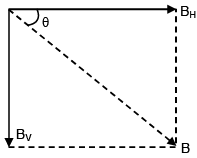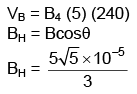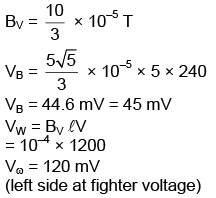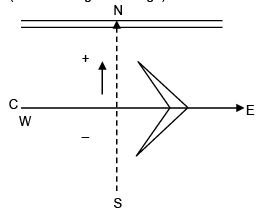Q 38. Consider a thin metallic sheet perpendicular to the plane of the paper moving with speed 'v' in a uniform magnetic field B going into the plane of the paper (see figure) . If charge densities σand σ2 are induced on the left and right surfaces, respectively, of the sheet then (ignore fringe effects)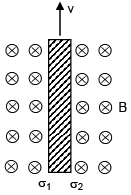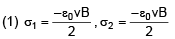(2) σ1 = σ20vB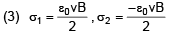(4) σ10vB, σ2 = -ε0vB
Ans:
(4)
Solution: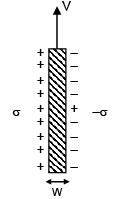direction of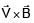is towards left therefore induced change dentition will be electric field =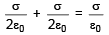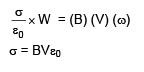Offer running on EduRev: Apply code STAYHOME200 to get INR 200 off on our premium plan EduRev Infinity!

,

,

,

,

,

,

,

,

,

,

,

,

,

,

,

,

,

,

,

,

,

;